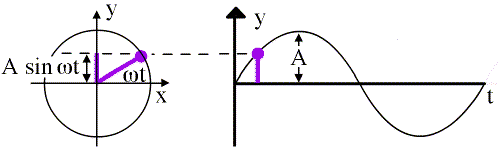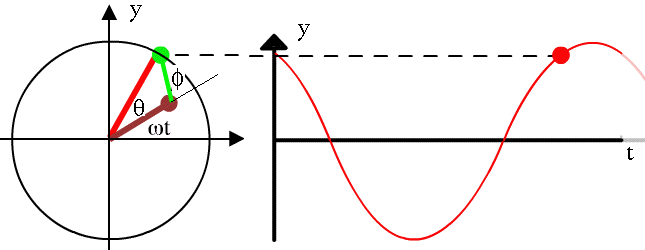Here we discuss phasor addition: a simple way of adding two or more simple harmonic oscillations. This is a background page to the multimedia chapter Interference.

### Mass on Spring: introducing phasors

In the chapter on Simple harmonic motion, we showed how it could be represented as a projection of uniform circular motion. Now let's be explicit:On this still from the animation above, the graph at right shows the displacement y of simple harmonic motion with amplitude A, angular frequency ω and zero initial phase:

y  =  A sin ωt.
If uniform circular motion has radius A, angular frequency ω and zero initial phase, then the angle between the radius (of length A) and the x axis is ωt as shown. The rotating arm here is called a phasor, which is a combination of vector and phase, because the direction of the vector (the angle it makes with the x axis) gives the phase.

### Two phasors with fixed phase

Let's see how useful this phasor representation is when we add simple harmonic motions having the same frequency but different phase.

Here we have the brown phasor with magnitude A and initial phase 0

y1  =  A sin ωt
and the green one with magnitude A and initial phase φ:
y2  =  B sin (ωt + φ).
We say y2 is ahead of y1 by φ or more commonly, y2 leads y1 by φ.From vector addition, we can see that the red phasor is the sum of the brown and the green ones. The amplitude and phase of the red phasor can then be obtained by trigonometry or geometry.

### Mixing two sounds

This film clip from the chapter Interference shows an example of the addition of two sine waves with varying amplitude and phase.

Both loudspeakers are being driven by the same oscillator and, assuming that the speakers are similar, they each output the same sound wave. The one that is closer to the camera and microphone will be both louder (larger amplitude) and ahead in phase. Adding such signals becomes a little more complicated.

### Constructive and desctructive interference

When one adds two simple harmonic motions having the same frequency and different phase, the resultant amplitude depends on their relative phase, on the angle between the two phasors. In this animation, we vary the relative phase to show the effect.

Here, the phasor diagram shows that the maximum amplitude occurs when the two are in phase: this is called constructive interference. The minimum sum arises when they are 180° out of phase, which is called destructive interference.

### What if the frequencies are different?

If one simple harmonic oscillation has angular frequency ω1 and the other angular frequency ω2, we could say that the second leads the first by a variable angle φ, where dφ/dt  =  ω2 − ω1. In general, this representation doesn't usually lead to substantial simplification. However, an interesting phenomenon arises when
f2/f1  =  ω21  =  m/n,     where m and n are small integers.
This is interesting, because it is also the condition for musical consonance. In the example below, the ratio is 3/2, which in music is the perfect fifth, one of the most harmonious consonances.

This animation shows how consonance may be demonstrated using Lissajous figures, in which one harmonic oscillation is plotted as the y coordinate and the other as the x coordinate. We have a separate page on Lissajous figures.

### Applications

Phasor sums are used to analyse interference and consonance in sound, the interference and diffraction of waves in general and in AC electricity.This work is licensed under a Creative Commons License.